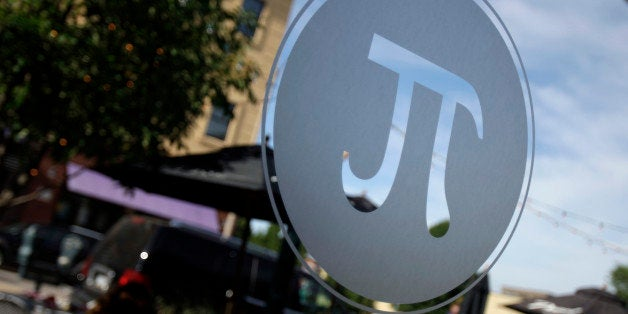# Pi's Top FiveThe symbol for Pi is seen on a window at Pi pizza Thursday, June 4, 2009, in St. Louis. (AP Photo/Jeff Roberson)

March 14th is not on the list of major holidays. This year, though, is particularly notable. Just one month after Valentine's Day, the first five digits of Pi are embedded in the date 3 14 15 -- that is a once in a century occurrence (only to be outdone in the year 1592, when the first 7 digits were embedded in the date). Considering the next three digits of Pi, 926, at 9:26 the first 8 digits will be embedded in date and time: 3 14 15 926.

It turns out this property is not completely unique to Pi -- a large class of numbers can find their digits similarly embedded into a particular day. But Pi is a special irrational number that has driven historically important results. To celebrate Pi Day, I am offering a list of the most important mathematical results related to Pi (in reverse chronological order of their discovery).

5) Squaring the Circle

It turns out the vast majority of numbers are actually transcendental. This means that a number is not the solution of any one of those tricky polynomials equations (ax^n+bx^(n-1)+...+c=0 where the coefficients are rational numbers) that drive so many algebra students nuts. The question of Pi's transcendence is tied to one of the great problems from antiquity, called "squaring the circle." By proving Pi is transcendental, Lindemann conclusively demonstrated that it was impossible to construct a square with the same area as a given circle using only a ruler and compass a finite number of times. At minimum for a number to be transcendental, it has to also be irrational, which means its decimal expansion never ends or repeats. Pi unlocked this truth.

4) The Normal Distribution and Area under e^-(x^2)

The normal distribution plays an essential role in statistics, science, and finance because of its connection to the Central Limit Theorem. It was constructed starting with the curve e^-(x^2). If you recall that the sum of the probabilities of all possible outcomes in a game of chance has to equal 1, that also means that for a function to be a probability distribution, its area has to be equal to 1. By showing the area under e^-(x^2) is 1/sqrt(π), we can adjust it and in doing so construct the standard normal distribution.

3. The Basel Problem: ∑(1/n^2) = (π^2)/6

Named for the hometown of Leonhard Euler (who solved it), the Basel Problem was originally proposed by mathematician and clergyman Pietro Mengolin. After the Harmonic Series and its alternating sister, the Basel Problem was the natural choice for an infinite series to study. Having survived almost 100 years of attempts by some of the world's best mathematical minds, it was known as one of the hardest mathematical problems of its day. Euler then went on to increase its importance by showing a deep connection between a generalized form of it and the prime numbers. Bernhard Riemann took it even further with his construction and work on the Zeta function. His hypothesis is probably the most important unsolved problem today.

2. Euler's Identity: e^(π*i) +1=0

Known as one of the most beautiful equations for its combination of the five most important numbers, the three core operations of math: addition, multiplication, and exponentiation (noting that subtraction and division are sisters of the first two), and nothing more, Euler's Identity shows math's capacity for wonder and simplicity amongst irrationality. Leonardo Da Vinci stated "simplicity is the ultimate sophistication"; Euler's identity is a prime example of this truth. Leonhard Euler went blind late in life, but left a powerful legacy that allows us to better understand the world we see and what we can't.

1. Area of a circle=πr^2

Discovered by Archimedes, it rivals the Pythagorean Theorem as the most famous mathematical equation. It is one of our first interactions with Pi in school, and one which is rarely forgotten. Archimedes also worked out the surface area and volume of a sphere.

So, whether you are a math geek, an aesthete or both, I hope you'll take the opportunity on Saturday morning to tip your cap to the number that contributes so much to our understanding of the world and our concept of beauty.